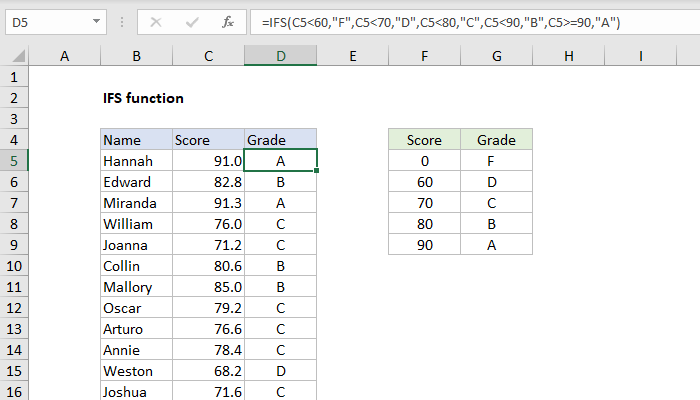Purpose
Test multiple conditions, return first true

Return value
Value corresponding with first TRUE result

Arguments
test1 - First logical test.
value1 - Result when test1 is TRUE.
test2, value2 - [optional] Second test/value pair.

Syntax
=IFS(test1, value1, [test2, value2], ...)

Structure
An IFS formula with 3 tests can be visualized like this:

=IFS(
test1,value1 // pair 1
test2,value2 // pair 2
test3,value3 // pair 3
)
A value is returned by IFS only when the previous test returns TRUE, and the first test to return TRUE "wins".  For better readability, you can add line breaks to an IFS formula as shown above.

Note: the IFS function does not provide an argument for a default value. See Example #3 below for a workaround.

Example #1 - grades, lowest to highest
In the example shown below, the IFS function is used to assign a grade based on a score. The formula in E5, copied down, is:
=IFS(C5<60,"F",C5<70,"D",C5<80,"C",C5<90,"B",C5>=90,"A")

Example #2 - rating, highest to lowest
In a simple rating system, a score of 3 or greater is "Good", a score between 2 and 3 is "Average", and anything below 2 is "Poor".  To assign these values with IFS, three conditions are used:
=IFS(A1>=3,"Good",A1>=2,"Average",A1<2,"Poor")

Example #3 - default value
The IFS function does not have a built-in default value to use when all conditions are FALSE. However, to provide a default value, you can enter TRUE as a final test, followed by a value to use as a default.
In the example below, a status code of 100 is "OK", a code of 200 is "Warning", and a code of 300 is "Error". Any other code value is invalid, so TRUE is provided as the final test, and "Invalid" is provided as a "default" value.
=IFS(A1=100,"OK",A1=200,"Warning",A1=300,"Error",TRUE,"Invalid")